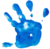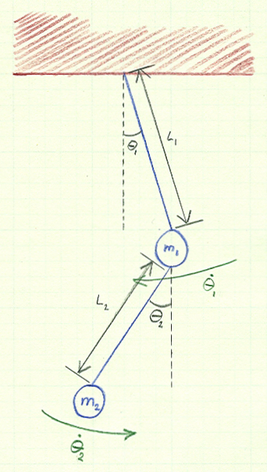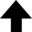To explain all nature is too difficult a task for any one man or even for any one age. 'Tis much better to do a little with certainty & leave the rest for others that come after you than to explain all things by conjecture without making sure of any thing.Isaac Newton, on the high standards of realism and precision in science

Hamiltonian systems are a very important class of dynamical systems. The Simple Pendulum is a well-known example. In general, the motion of Hamiltonian systems is very complex, but seems to fall into three classes: integrable, chaotic, and mixed. The double pendulum is an example of a dynamical system that can exhibit chaotic behavior. This system consists of two point masses $m_1$ and $m_2$, suspended by rigid weightless rods of lengths $L_1$ and $L_2$, respectively. (An analytical treatment of this problem is given in my classical mechanics section.) Because there is no friction, this system is clearly an example of a Hamiltonian system.

Reference• Neumann, Erik. "Double Pendulum Physics Simulation." My Physics Lab.

Hamiltonian Chaos

This program solves the coupled equations of motion of a double pendulum to simulate chaos for large amplitude oscillations.
$\displaystyle{ \ddot{\theta_1} = \frac{-g (2 m_1 + m_2) \sin \theta_1 - m_2 g \sin (\theta_1 - 2 \theta_2) - 2 \sin (\theta_1 - \theta_2) m_2 \left[ \dot{\theta}_2^2 L_2 + \dot{\theta}_1^2 L_1 \cos (\theta_1 - \theta_2) \right] }{L_1 \left[ 2 m_1 + m_2 - m_2 \cos (2 \theta_1 - 2 \theta_2) \right] } }$ $\displaystyle{ \ddot{\theta_2} = \frac{2 \sin (\theta_1 - \theta_2) \left[ \dot{\theta}_1^2 L_1 (m_1 + m_2) + g (m_1 + m_2) \cos \theta_1 + \dot{\theta}_2^2 L_2 m_2 \cos(\theta_1 - \theta_2) \right] }{L_2 \left[ 2 m_1 + m_2 - m_2 \cos (2 \theta_1 - 2 \theta_2) \right] } }$

Upper Pendulum

 Initial angle $\theta_1$: rad0.0 ≤ $\theta_1$ ≤ 1.6 rad Initial angular velocity $\dot{\theta_1}$: rad/sec0.0 ≤ $\dot{\theta_1}$ ≤ $\pi$ rad/sec Length of pendulum $L_1$: m0.1 ≤ $L_1$ ≤ 2.0 m Mass of pendulum bob $m_1$: kg0.1 ≤ $m_1$ ≤ 2.0 kg

Lower Pendulum

 Initial angle $\theta_2$: rad0.0 ≤ $\theta_2$ ≤ 1.6 rad Initial angular velocity $\dot{\theta_2}$: rad/sec0.0 ≤ $\dot{\theta_2}$ ≤ $\pi$ rad/sec Length of pendulum $L_2$: m0.1 ≤ $L_2$ ≤ 2.0 m Mass of pendulum bob $m_2$: kg0.1 ≤ $m_2$ ≤ 2.0 kg Steps:
0%Current data point: